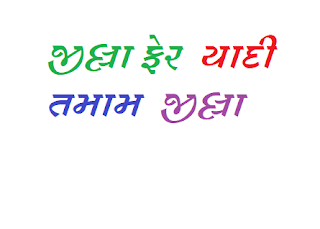## 23 November 2015

### Script for Useful maths tricks for competitive exam

How to find a CUBE ROOT of number

13824
Step 1: Now to find the unit place of the cube root always remember the following points
1.If the Last digit of a cube root is 8 than the unit digit will be 2.
2.If the Last digit of a cube root is 2 than the unit digit will be 8.
3.If the Last digit of a cube root is 3 than the unit digit will be 7.
4.If the Last digit of a cube root is 7 than the unit digit will be 3.
5.If the Last digit of a cube root is other than 2,3,7,8 than put the same number as the unit digit.
Now the unit digit will be 4

Step 2: Now strike the last 3 digits of the given number.
13824
Step 3: Now find the nearest square of the first 2 digit from the left
Now the nearest cube is 8
Step 4now 8 is the cube root of 2
Therefore the ten’s place digit is 2
So the answer will be 24
Like this get many tricks of maths and score in the competitive exam with kachhua...
Kachhua can help you to prepare well in competitive exam by providing online material , video lectures and regular online tests(provided with Auto result and Daily solution)...

click the below link for preparation of Mathematics with kachhua..

Maths (Only Daily Test)

Do Online preparation of std. 6 to 10 on your mobile or PC...
Click the below links and fill the form for online preparation of std. 6 to 10...

Standard 6
Standard 7